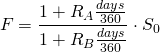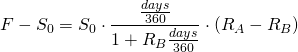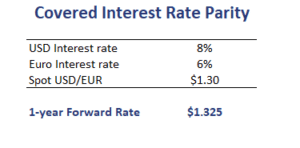# Covered Interest Rate Parity

What is the covered interest rate parity? Covered refers to the fact that this kind of interest rate parity will hold if arbitrage is possible. When arbitrage is not possible or capital flows are restricted, then covered interest parity will not necessarily hold. In that case, the uncovered interest rate parity is more useful and should be used. Using the covered interest rate parity, we can calculate the forward discount or forward premium of a currency forward contract.

On this page, we discuss the covered rate parity formula, the forward discount/premium formula and illustrate both formulas using a numerical example. The Excel spreadsheet used can be downloaded at the bottom of the page.

## Covered interest rate parity formula

As we already discussed in the introduction, “covered” means that this kind of interest rate parity will generally hold thanks to arbitrage activity. When covered rate parity holds, then any forward premium or discount exactly offsets differences in interest rates. In that case, an investor will earn the same return investing in either currency.

The covered interest rate formula looks as followswhere F is the forward rate (in A/B), S0 is the spot rate (in A/B), days is the number of days in the forward contract, RA (RB) is the interest rate for currency A (B).

We can calculate the currency forward premium or discount as follows## Covered Interest rate parity example

Let’s consider an example. The following table illustrates the use of the formula using a numerical example. In particular, we consider a he covered interest rate arbitrage example.

If the covered rate parity does not hold, a covered interest rate arbitrage is possible if it is not restricted somehow.The covered interest arbitrage calculator is available as an Excel at the bottom of the page.

## Summary

We discussed the covered rate parity and the currency forward premium or discount based on the covered rate parity formula.